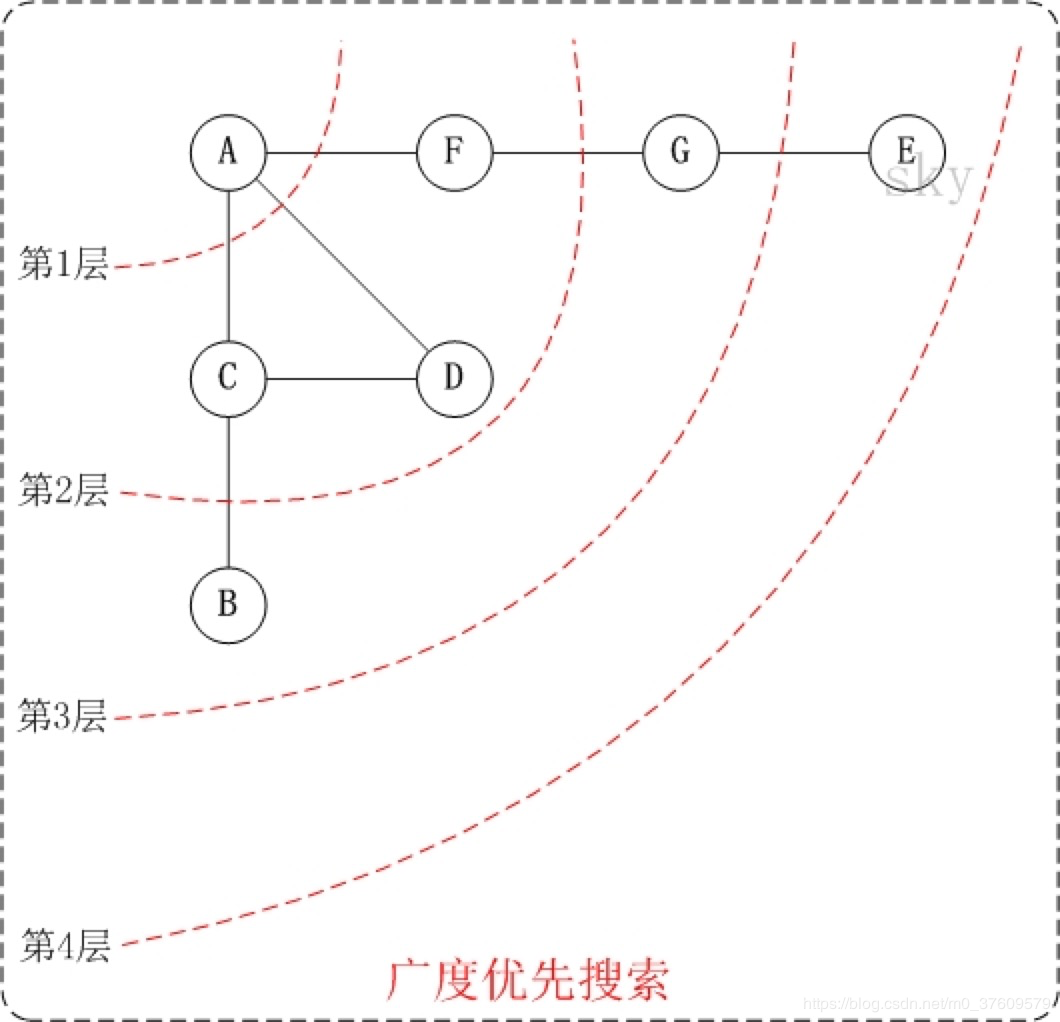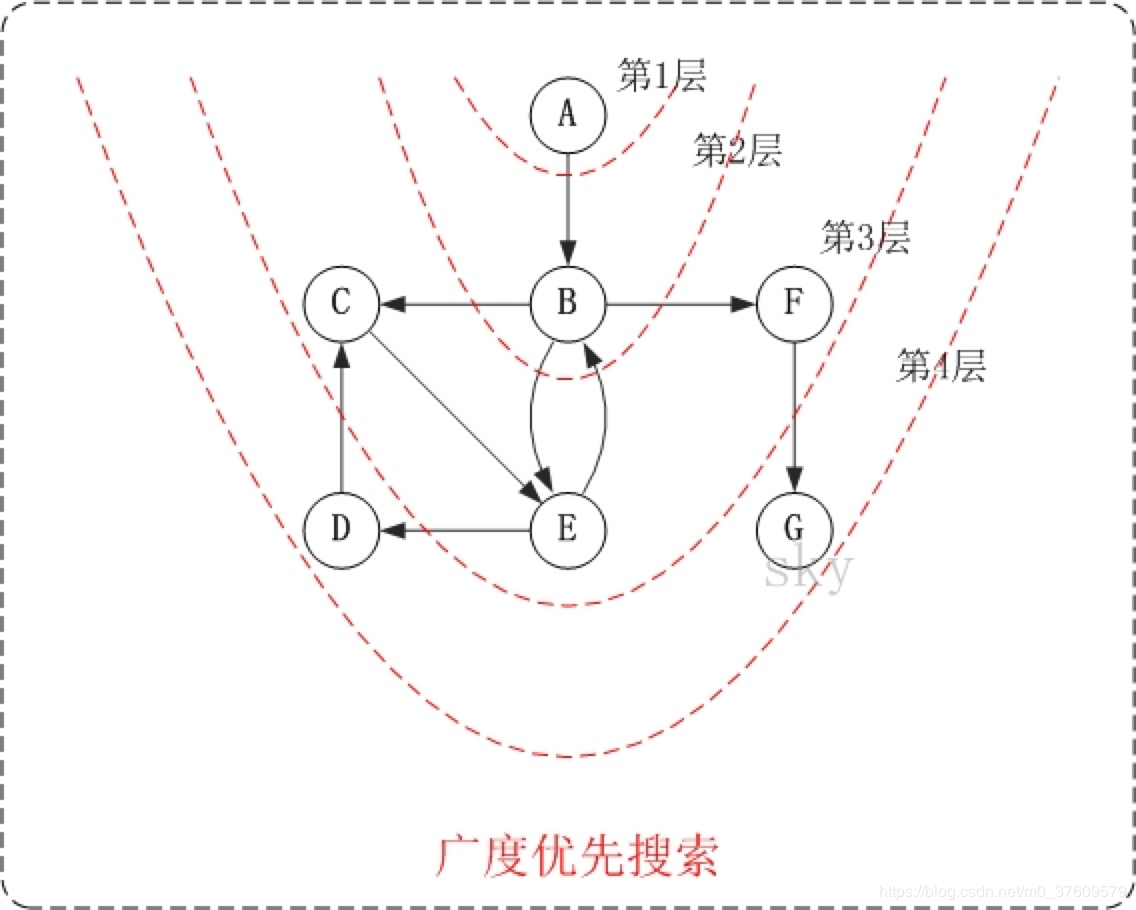• 随笔 - 43
• 文章 - 1
• 评论 - 3
• 引用 - 0

# 二、广度优先搜索图解

### 1.无向图的广度优先搜索1. 第1步：访问A。
2. 第2步：依次访问C,D,F。 在访问了A之后，接下来访问A的邻接点。前面已经说过，在本文实现中，顶点ABCDEFG按照顺序存储的，C在"D和F"的前面，因此，先访问C。再访问完C之后，再依次访问D,F。
3. 第3步：依次访问B,G。在第2步访问完C,D,F之后，再依次访问它们的邻接点。首先访问C的邻接点B，再访问F的邻接点G。
4. 第4步：访问E。 在第3步访问完B,G之后，再依次访问它们的邻接点。只有G有邻接点E，因此访问G的邻接点E。

### 2.有向图的广度优先搜索1. 第1步：访问A。
2. 第2步：访问B。
3. 第3步：依次访问C,E,F。 在访问了B之后，接下来访问B的出边的另一个顶点，即C,E,F。前面已经说过，在本文实现中，顶点ABCDEFG按照顺序存储的，因此会先访问C，再依次访问E,F。
4. 第4步：依次访问D,G。在访问完C,E,F之后，再依次访问它们的出边的另一个顶点。还是按照C,E,F的顺序访问，C的已经全部访问过了，那么就只剩下E,F；先访问E的邻接点D，再访问F的邻接点G。

# 三、代码实现

/**
* 图的广度优先遍历算法
*/
private void boardFirstSearch(int i) {
System.out.println("访问到了：" + i + "顶点");
isVisited[i] = true;

while (queue.size() > 0) {
int w = queue.removeFirst().intValue();
int n = getFirstNeighbor(w);
while (n != -1) {
if (!isVisited[n]) {
System.out.println("访问到了：" + n + "顶点");
isVisited[n] = true;
}
n = getNextNeighbor(w, n);
}
}
}

# 四、图的DFS和BFS完整代码

import java.util.LinkedList;

public class Graph {

private int vertexSize; // 顶点数量
private int[] vertexs; // 顶点数组
private int[][] matrix; // 包含所有顶点的数组
// 路径权重
// 0意味着顶点自己到自己，无意义
// MAX_WEIGHT也意味着到目的顶点不可达
private static final int MAX_WEIGHT = 1000;
private boolean[] isVisited; // 某顶点是否被访问过

public Graph(int vertextSize) {
this.vertexSize = vertextSize;
matrix = new int[vertextSize][vertextSize];
vertexs = new int[vertextSize];
for (int i = 0; i < vertextSize; i++) {
vertexs[i] = i;
}
isVisited = new boolean[vertextSize];
}

/**
* 获取指定顶点的第一个邻接点
*
* @param index
*          指定邻接点
* @return
*/
private int getFirstNeighbor(int index) {
for (int i = 0; i < vertexSize; i++) {
if (matrix[index][i] < MAX_WEIGHT && matrix[index][i] > 0) {
return i;
}
}
return -1;
}

/**
* 获取指定顶点的下一个邻接点
*
* @param v
*          指定的顶点
* @param index
*          从哪个邻接点开始
* @return
*/
private int getNextNeighbor(int v, int index) {
for (int i = index+1; i < vertexSize; i++) {
if (matrix[v][i] < MAX_WEIGHT && matrix[v][i] > 0) {
return i;
}
}
return -1;
}

/**
* 图的深度优先遍历算法
*/
private void depthFirstSearch(int i) {
isVisited[i] = true;
int w = getFirstNeighbor(i);
while (w != -1) {
if (!isVisited[w]) {
// 需要遍历该顶点
System.out.println("访问到了：" + w + "顶点");
depthFirstSearch(w); // 进行深度遍历
}
w = getNextNeighbor(i, w); // 第一个相对于w的邻接点
}
}

/**
* 图的广度优先遍历算法
*/
private void boardFirstSearch(int i) {
System.out.println("访问到了：" + i + "顶点");
isVisited[i] = true;

while (queue.size() > 0) {
int w = queue.removeFirst().intValue();
int n = getFirstNeighbor(w);
while (n != -1) {
if (!isVisited[n]) {
System.out.println("访问到了：" + n + "顶点");
isVisited[n] = true;
}
n = getNextNeighbor(w, n);
}
}
}

public static void main(String[] args) {
Graph graph = new Graph(9);

// 顶点的矩阵设置
int[] a1 = new int[] { 0, 10, MAX_WEIGHT, MAX_WEIGHT, MAX_WEIGHT, 11, MAX_WEIGHT, MAX_WEIGHT, MAX_WEIGHT };
int[] a2 = new int[] { 10, 0, 18, MAX_WEIGHT, MAX_WEIGHT, MAX_WEIGHT, 16, MAX_WEIGHT, 12 };
int[] a3 = new int[] { MAX_WEIGHT, MAX_WEIGHT, 0, 22, MAX_WEIGHT, MAX_WEIGHT, MAX_WEIGHT, MAX_WEIGHT, 8 };
int[] a4 = new int[] { MAX_WEIGHT, MAX_WEIGHT, 22, 0, 20, MAX_WEIGHT, 24, 16, 21 };
//int[] a4 = new int[] { MAX_WEIGHT, MAX_WEIGHT, 22, 0, 20, MAX_WEIGHT, MAX_WEIGHT, 16, 21 };
int[] a5 = new int[] { MAX_WEIGHT, MAX_WEIGHT, MAX_WEIGHT, 20, 0, 26, MAX_WEIGHT, 7, MAX_WEIGHT };
int[] a6 = new int[] { 11, MAX_WEIGHT, MAX_WEIGHT, MAX_WEIGHT, 26, 0, 17, MAX_WEIGHT, MAX_WEIGHT };
int[] a7 = new int[] { MAX_WEIGHT, 16, MAX_WEIGHT, 24, MAX_WEIGHT, 17, 0, 19, MAX_WEIGHT };
//int[] a7 = new int[] { MAX_WEIGHT, 16, MAX_WEIGHT, MAX_WEIGHT, MAX_WEIGHT, 17, 0, 19, MAX_WEIGHT };
int[] a8 = new int[] { MAX_WEIGHT, MAX_WEIGHT, MAX_WEIGHT, 16, 7, MAX_WEIGHT, 19, 0, MAX_WEIGHT };
int[] a9 = new int[] { MAX_WEIGHT, 12, 8, 21, MAX_WEIGHT, MAX_WEIGHT, MAX_WEIGHT, MAX_WEIGHT, 0 };

graph.matrix = a1;
graph.matrix = a2;
graph.matrix = a3;
graph.matrix = a4;
graph.matrix = a5;
graph.matrix = a6;
graph.matrix = a7;
graph.matrix = a8;
graph.matrix = a9;

graph.depthFirstSearch(0);
//graph.boardFirstSearch(0);
}

}

# 五、总结

• 广度优先遍历表示把每一层都遍历完才能遍历下一层
• 我们来思考：假设v0有3个邻接点，v1 v2 v3
• 我们访问v0后，然后访问v1 v2 v3。完毕后我们要从v1开始遍历它的邻接点，接着从v2开始遍历它的邻接点，最后是从v3开始遍历它的邻接点。
• 也就是说，3个邻接点访问完后。我们要回过头逐个遍历它们的邻接点。这一点我觉得要用个容器把它们顺序存储下来。然后每次从容器首部取出一个顶点开始遍历。这里我想到LinkedList，因为它适合增删。而且这里不需要遍历集合。
• 我们可以把第一个顶点放进集合，然后while(!queue.isEmpty())while(queue.size() > 0)都行。开始循环。
• 然后取出并删除集合中第一个顶点元素的第一个邻接点。对这个顶点进行访问，
• 如果该顶点未访问过，就访问！然后将该顶点放入集合。
• 如果该顶点已访问过，就找该顶点的下一个邻接点。posted on 2019-09-15 11:23  十步杀一人_沧海君  阅读(34)  评论(0编辑  收藏